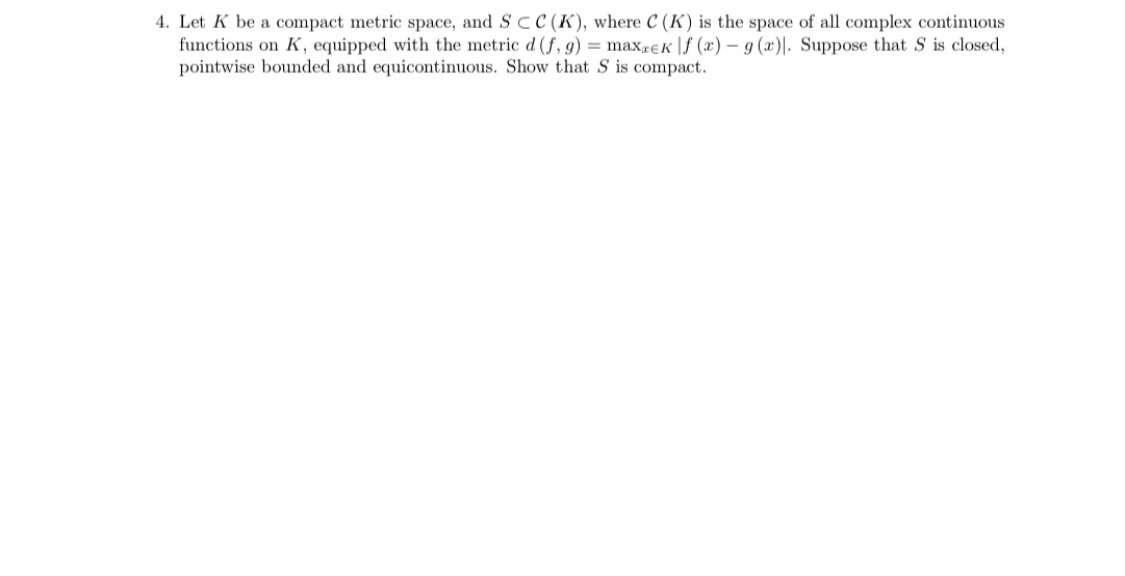# 4. Let K be a compact metric space, and Scc (K), where C (K) is the space of all complex continuousfunctions on K, equipped with the metric d (f,9)maxreK f() (x)|. Suppose that S is closed,pointwise bounded and equicontinuous. Show that S is compact.

Questionhelp_outlineImage Transcriptionclose4. Let K be a compact metric space, and Scc (K), where C (K) is the space of all complex continuous functions on K, equipped with the metric d (f,9)maxreK f() (x)|. Suppose that S is closed, pointwise bounded and equicontinuous. Show that S is compact. fullscreen
check_circleExpert Solution
Step 1

To establish the compactness of the set S in the given topology

Step 2

Recall the  basic facts about compactness in metric spaces

Step 3

In view o the facts (1),(2) (3), it suffices to prove ...

### Want to see the full answer?

See Solution

#### Want to see this answer and more?

Solutions are written by subject experts who are available 24/7. Questions are typically answered within 1 hour*

See Solution
*Response times may vary by subject and question
Tagged in

### Math